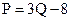Chapter 5, Problem 36P### Principles of Economics 2e

2nd Edition
Steven A. Greenlaw; David Shapiro
ISBN: 9781947172364

#### Solutions

Chapter
Section### Principles of Economics 2e

2nd Edition
Steven A. Greenlaw; David Shapiro
ISBN: 9781947172364
Textbook Problem

# The equation for a supply curve isWhat is the elasticity in moving from a price of 4 to a price of 7?

To determine

To calculate: the elasticity of supply when price moves from $4 to$7.

Explanation

We will first find the quantity supplied at price $4. P= 3Q - 84 = 3Q - 83Q = 12Q = 4 units at P =$4

Now, we will find quantity supplied at price $7. P = 3Q - 87 = 3Q - 815 = 3QQ = 5 units at P =$7

Now, we can calculate the elasticity of suppl

### Still sussing out bartleby?

Check out a sample textbook solution.

See a sample solution

#### The Solution to Your Study Problems

Bartleby provides explanations to thousands of textbook problems written by our experts, many with advanced degrees!

Get Started

#### Find more solutions based on key concepts